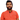# Learn Pain LessHomeOur TeamContact
Python
How to understand modulus % in pythonPawneshwer Gupta
March 28, 2023
1 min

01
What is modulus % in Python?
02
Examples of using modulus in Python
03
ConclusionUnderstanding modulus % in Python can be a challenging concept to grasp, yet it’s essential for becoming an accomplished programmer. In this article, we’ll define what modulus % is, explain its functionality within Python, and provide some examples to make things clearer for you.

## .css-1qh5hbx{box-sizing:border-box;margin:0;min-width:0;display:block;color:var(--theme-ui-colors-heading,#2d3748);font-weight:bold;-webkit-text-decoration:none;text-decoration:none;margin-bottom:1rem;font-size:1.5rem;position:relative;}What is modulus % in Python?

Python’s modulus operator % returns the result of dividing two numbers by 1. For instance, 5 % 2 would return 1, since 2 goes into 5 twice with a remainder of 1. This operator can be applied to both integers and floating-point numbers alike; even negative numbers can be divided by this operator.

Modulus is commonly used in programming to check if a number is even or odd. An even number will be evenly divisible by 2, meaning the remainder when dividing by 2 will be 0. Conversely, an odd number would yield a remainder of 1.

## Examples of using modulus in Python

Let’s examine some examples to understand modulus in Python. Let’s start with an easy example of checking if a number is even or odd:

`.css-1baulvz{display:inline-block;}x = 7if x % 2 == 0:    print("x is even")else:    print("x is odd")`

In this example, we set x to 7 and then used the modulus operator to check if it is even or odd. Because 7 % 2 equals 1, the else statement executes and returns “x is odd”.

Another common use of modulus in programming is to wrap around an index in a list or array. For instance, let’s say we have a list of numbers and want to loop through it circularly. We can use modulus to wrap around the index:

`numbers = [1, 2, 3, 4, 5]index = 0for i in range(10):    print(numbers[index % len(numbers)])    index += 1`

In this example, we define a list of numbers and an index variable, then loop through it 10 times. We use modulus to wrap around the index when it reaches its end in the list; this allows us to loop through in circular fashion and print out each number individually.

## Conclusion

Understanding modulus % in Python is essential for any programmer who aspires to write efficient and effective code. By using the modulus operator, you can perform useful tasks such as checking if a number is even or odd and wrapping around an index of a list. We hope this article has provided you with sufficient understanding of modulus % in Python.

We'll send you the best of our blog just once a month. We promise.

Question

## Software Developer

Pawneshwer Gupta works as a software engineer who is enthusiastic in creating efficient and innovative software solutions.

Python
Flutter
Laravel
NodeJS

## Related PostsWhat is Self in Python: A Comprehensive Guide
March 24, 2023
5 min